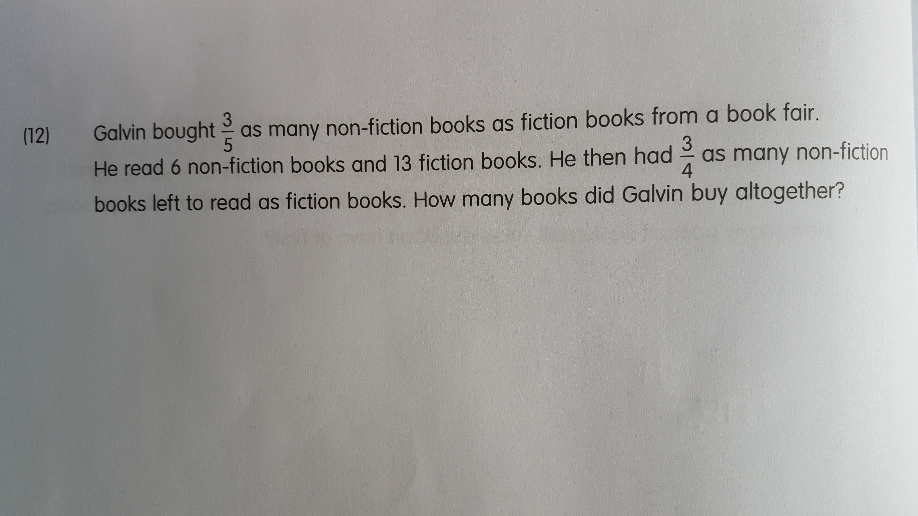# QuestionLet the original number of books bought be 8B

Let the total number of books left to read be 7X

3B – 6 = 3X

5B – 13 = 4X

X = B – 2

X +2 = B

3X + 4X = 3B – 6 + 5B – 13
7X = 8B – 19
7X + 19 = 8B
7X + 19 = 8(X+2) = 8x + 16
8X – 7X = 19-16
X = 3

8B = 7(3) + 19 = 40

Test for correct answer

B = 5

Original no. of  non fiction books = 3B = 15
Original no. of fiction books = 5B = 25

15 – 6 = 9

25 – 13 = 12

9/12 is a ratio of 3/4

Therefore, the answer is proven.

0 Replies 0 Likes

Let the original number of books bought be 8B

Let the total number of books left to read be 7X

3B – 6 = 3X

5B – 13 = 4X

X = B – 2

X +2 = B

3X + 4X = 3B – 6 + 5B – 13
7X = 8B – 19
7X + 19 = 8B
7X + 19 = 8(X+2) = 8x + 16
8X – 7X = 19-16
X = 3

8B = 7(3) + 19 = 40

Test for correct answer

B = 5

Original no. of  non fiction books = 3B = 15
Original no. of fiction books = 5B = 25

15 – 6 = 9

25 – 13 = 12

9/12 is a ratio of 3/4

Therefore, the answer is proven.

0 Replies 0 Likes